NCERT Solutions: Polynomials (Exercise 2.4)

# Polynomials (Exercise 2.4) NCERT Solutions - Class 10 Mathematics by VP Classes

Exercise 2.4
Ques 1: Verify that the numbers given alongside of the cubic polynomials below are their zeroes. Also verify the relationship between the zeroes and the coefficients in each case:
(i) 2x3 + x2 - 5x + 2;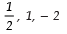(ii) x3 - 4x2 + 5x - 2; 2, 1, 1
Sol: (i) ∵ p (x) = 2x3 + x2 - 5x + 2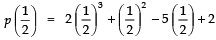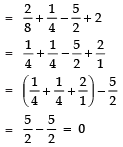⇒ 1/2 is a zero of p(x).
Again,
p (1) = 2 (1)3 + (1)2 - 5 (1) + 2
= 2 + 1 - 5 + 2
= (2 + 2 + 1) - 5
= 5 - 5 = 0
⇒ 1 is a zero of p (x).
Also p (- 2) = 2 (- 2)3 + (- 2)2 - 5 (- 2) + 2
= 2(- 8) + (4) + 10 + 2
= - 16 + 4 + 10 + 2
= - 16 + 16 = 0
⇒ -2 is a zero of p (x).
Relationship
∵ p(x) = 2x3 + x2 - 5x + 2
∴ Comparing it with ax3 + bx2 + cx + d, we have :
a = 2, b = 1, c = - 5 and d = 2
Also 1/2 , 1 and - 2 are the zeroes of p(x)
Let α = 1/2 , β = 1 and γ = - 2
∴ α + β +γ =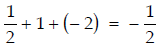Sum of the zeroes =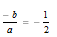⇒ α + β + γ = -b/a
Sum of product of zeroes taken in pair: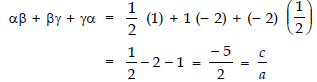Product of zeroes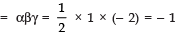Also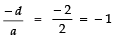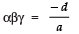Thus, the relationship between the co-efficients and the zeroes of p (x) is verified.
(ii) Here, p(x) = x3 - 4x2 + 5x - 2
∴ p (2) = (2)3 - 4 (2)2 + 5 (2) - 2
= 8 - 16 + 10 - 2
= 18 - 18 = 0
⇒ 2 is a zero of p(x)
Again p (1) = (1)3 - 4 (1)2 + 5 (1) - 2
= 1 - 4 + 5 - 2
= 6 - 6 = 0
⇒ 1 is a zero of p(x).
∴ 2, 1, 1 are zeroes of p(x).
Now,
Comparing p (x) = x3 - 4 (x2) + 5x - 2 with ax3 + bx2 + cx + d = 0, we have
a = 1, b = - 4, c = 5 and d = - 2
∵ 2, 1, and 1 are the zeroes of p (x)
∴ Let α = 2
β = 1
γ = 1
Relationship, α + β + γ = 2 + 1 + 1 = 4
Sum of the zeroes =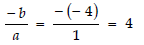⇒ α + β + γ = (-b/a)
Sum of product of zeroes taken in pair:
αβ + βγ + γα = 2 (1) + 1 (1) + 1 (2)
= 2 + 1 + 2 = 5
and = c/a = 5/1 = 5
⇒ αβ + βγ + γα = c/a
Product of zeroes = αβγ = (2) (1) (1) = 2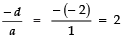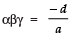Thus, the relationship between the zeroes and the co-efficients of p(x) is verified.

Ques 2: Find the cubic polynomial with the sum, sum of the products of its zeroes taken two at a time and the product of its zeroes as 2, - 7, - 14 respectively.
Sol: Let the required cubic polynomial be ax3 + bx2 + cx + d and its zeroes be a, b and g.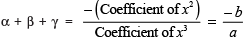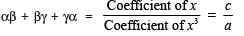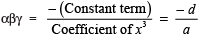Since,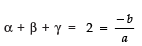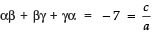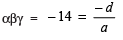If a = 1, then -b/a = 2 ⇒ b = - 2
c/a = - 7 ⇒ c = - 7
-d/a = - 14 ⇒ d = 14
∴ The required cubic polynomial
= 1x3 + (-2) x2 + (-7) x + 14
= x3 - 2x2 - 7x + 14

Ques 3: If the zeroes of the polynomial x3 - 3x2 + x + 1 are a - b, a and a + b then find ‘a’ and ‘b’.
Sol: We have
p (x) = x3 - 3x+ x + 1
comparing it with Ax3 + Bx2 + Cx + D.
We have
A = 1, B = - 3, C = 1 and D = 1
∵ It is given that (a - b), a and (a + b) are the zeroes of the polynomial.
∴ Let, α = (a - b)
β = a
and γ = (a + b)
∴ α + β + γ =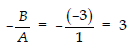⇒ (a - b) + a + (a + b) = 3
⇒ 3a = 3
⇒ a = 3/3 = 1
Again, αβγ = -D/A = -1
⇒ (a - b) × a × (a + b) = - 1
⇒ (1 - b) × 1 × (1 + b) = - 1 [∵ a = 1, proved above]
⇒ 1 - b2 = - 1
⇒ b2 = 1 + 1 = 2
⇒ b =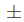√2
Thus, a = 1 and b =.√2

Ques 4: If two zeroes of the polynomial x4 - 6x3 - 26x2 + 138x - 35 are 23, find other zeroes.
Sol: Here, p(x) = x4 - 6x3 - 26x2 + 138x - 35.
∵ Two of the zeroes of p (x) are :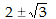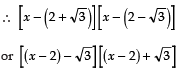or (x - 2)2 - (√3)2
or (x2 + 4 - 4x) - 3
or x2 - 4x + 1 is a factor of p(x).
Now, dividing p (x) by x2 - 4x + 1, we have :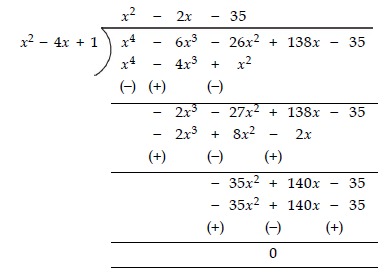∴ (x- 4x + 1) (x2 - 2x - 35) = p (x)
⇒ (x2 - 4x + 1) (x - 7) (x + 5) = p (x)
i.e., (x - 7) and (x + 5) are other factors of p(x).
∴ 7 and - 5 are other zeroes of the given polynomial.

Ques 5: If the polynomial x4 - 6x3 + 16x2 - 25x + 10 is divided by another polynomial x2 - 2x + k, the remainder comes out to be (x + α), find k and α.
Sol: Applying the division algorithm to the polynomials x4 - 6x3 + 16x2 - 25x + 10 and
x2 - 2x + k, we have: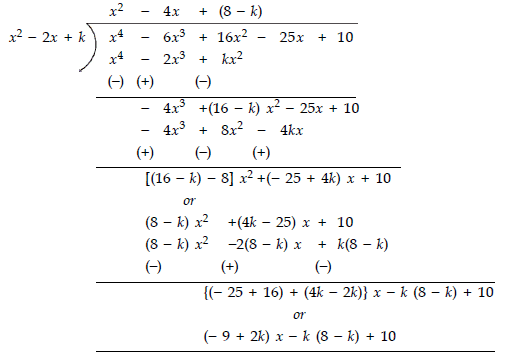∴ Remainder = (2k - 9) x - k (8 - k) + 10
But the remainder = x + α
Therefore, comparing them, we have :
2k - 9 = 1
⇒ 2k = 1 + 9 = 10
⇒ k = 10/2 = 5
and
α = - k (8 - k) + 10
= - 5 (8 - 5) + 10
= - 5 (3) + 10
= - 15 + 10
= - 5
Thus, k = 5 and α = - 5

The document Polynomials (Exercise 2.4) NCERT Solutions | Class 10 Mathematics by VP Classes is a part of the Class 10 Course Class 10 Mathematics by VP Classes.
All you need of Class 10 at this link: Class 10

132 docs

## FAQs on Polynomials (Exercise 2.4) NCERT Solutions - Class 10 Mathematics by VP Classes

 1. What are polynomials?Ans. Polynomials are algebraic expressions that consist of variables, coefficients, and exponents. They are made up of terms, which are separated by addition or subtraction operators. The highest exponent in a polynomial determines its degree.
 2. How can we classify polynomials based on their degrees?Ans. Polynomials can be classified as constant (degree 0), linear (degree 1), quadratic (degree 2), cubic (degree 3), quartic (degree 4), and so on. The degree of a polynomial is determined by the highest exponent of the variable.
 3. What is the Remainder Theorem?Ans. The Remainder Theorem states that if a polynomial P(x) is divided by a linear polynomial (x - a), then the remainder obtained is equal to P(a). In other words, if we substitute the value of 'a' into the polynomial, the remainder will be the same as the result obtained by dividing the polynomial by (x - a).
 4. How can we find the zeroes of a polynomial?Ans. The zeroes of a polynomial are the values of 'x' for which the polynomial becomes zero. We can find the zeroes by equating the polynomial to zero and solving the equation. This can be done by factorizing the polynomial or by using methods like the Remainder Factor Theorem or the synthetic division method.
 5. What is the Fundamental Theorem of Algebra?Ans. The Fundamental Theorem of Algebra states that every polynomial equation of degree 'n' has exactly 'n' complex roots, considering multiple roots as separate roots. This theorem guarantees that any polynomial equation can be solved, either exactly or approximately, by finding its roots.

## Class 10 Mathematics by VP Classes

132 docsExplore Courses for Class 10 examSignup to see your scores go up within 7 days! Learn & Practice with 1000+ FREE Notes, Videos & Tests.
10M+ students study on EduRev
Track your progress, build streaks, highlight & save important lessons and more!
Related Searches

,

,

,

,

,

,

,

,

,

,

,

,

,

,

,

,

,

,

,

,

,

;